This article was published as a part of the Data Science Blogathon.

## What Is Logistic Regression?

This article assumes that you possess basic knowledge and understanding of Machine Learning Concepts, such as Target Vector, Features Matrix, and related terms.

Logistic Regression- Probably one of the most interesting Supervised Machine Learning Algorithms in Machine Learning. Despite having “Regression” in its name, Logistic Regression is a popularly used Supervised Classification Algorithm. Logistic Regression, along with its related cousins viz. Multinomial Logistic Regression, grant us the ability to predict whether an observation belongs to a certain class using an approach that is straightforward, easy-to-understand, and follows.Source: DZone

Logistic Regression in its base form (by default) is a Binary Classifier. This means that the target vector may only take the form of one of two values. In the Logistic Regression Algorithm formula, we have a Linear Model, e.g., β0 + β1x, that is integrated into a Logistic Function (also known as a Sigmoid Function). The Binary Classifier formula that we have at the end is as follows: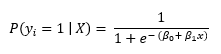Where:

• P(yi = 1 | X) is the probability of the ith observations target value, yi belonging to class 1.
• Β0 and β1 are the parameters that are to be learned.
• e represents Euler’s Number.

## Main Aim of Logistic Regression Formula.

The Logistic Regression formula aims to limit or constrain the Linear and/or Sigmoid output between a value of 0 and 1. The main reason is for interpretability purposes, i.e., we can read the value as a simple Probability; Meaning that if the value is greater than 0.5 class one would be predicted, otherwise, class 0 is predicted.## Python Implementation.

We shall now look at the implementation of the Python Programming Language. For this exercise, we will be using the Ionosphere dataset which is available for download from the UCI Machine Learning Repository.

```# We begin by importing the necessary packages
# to be used for the Machine Learning problem

import pandas as pd
import numpy as np
from sklearn.linear_model import LogisticRegression
from sklearn.preprocessing import StandardScaler

# We read the data into our system using Pandas'
# 'read_csv' method. This transforms the .csv file
# into a Pandas DataFrame object.

# We configure the display settings of the
# Pandas DataFrame.

pd.set_option('display.max_rows', 10000000000)
pd.set_option('display.max_columns', 10000000000)
pd.set_option('display.width', 95)

# We view the shape of the dataframe. Specifically
# the number of rows and columns present.
print('This DataFrame Has %d Rows and %d Columns'%(dataframe.shape))```

Output to the above code would be as follows (the shape of the dataframe):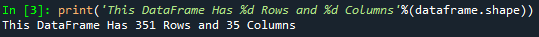```# We print the first five rows of our dataframe.

Output to the above code will be seen as follows (below output is truncated):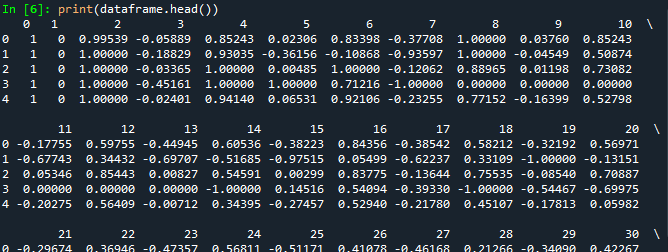```# We isolate the features matrix from the DataFrame.
features_matrix = dataframe.iloc[:, 0:34]

# We isolate the target vector from the DataFrame.
target_vector = dataframe.iloc[:, -1]

# We check the shape of the features matrix, and target vector.
print('The Features Matrix Has %d Rows And %d Column(s)'%(features_matrix.shape))
print('The Target Matrix Has %d Rows And %d Column(s)'%(np.array(target_vector).reshape(-1, 1).shape))```

Output for the shape of our Features Matrix and Target Vector would be as below: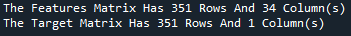```# We use scikit-learn's StandardScaler in order to
# preprocess the features matrix data. This will
# ensure that all values being inputted are on the same
# scale for the algorithm.

features_matrix_standardized = StandardScaler().fit_transform(features_matrix)```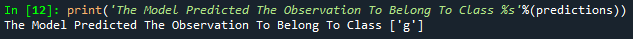```# We create an instance of the LogisticRegression Algorithm
# We utilize the default values for the parameters and
# hyperparameters.

algorithm = LogisticRegression(penalty='l2', dual=False, tol=1e-4,
C=1.0, fit_intercept=True,
intercept_scaling=1, class_weight=None,
random_state=None, solver='lbfgs',
max_iter=100, multi_class='auto',
verbose=0, warm_start=False, n_jobs=None,
l1_ratio=None)

# We utilize the 'fit' method in order to conduct the
# training process on our features matrix and target vector.

Logistic_Regression_Model = algorithm.fit(features_matrix_standardized, target_vector)```
```# We create an observation with values, in order
# to test the predictive power of our model.

observation = [[1, 0, 0.99539, -0.05889, 0.8524299999999999, 0.02306,
0.8339799999999999, -0.37708, 1.0, 0.0376,
0.8524299999999999, -0.17755, 0.59755, -0.44945, 0.60536,
-0.38223, 0.8435600000000001, -0.38542, 0.58212, -0.32192,
0.56971, -0.29674, 0.36946, -0.47357, 0.56811, -0.51171,
0.41078000000000003, -0.46168000000000003, 0.21266, -0.3409,
0.42267, -0.54487, 0.18641, -0.453]]```
```# We store the predicted class value in a variable
# called 'predictions'.

predictions = Logistic_Regression_Model.predict(observation)```
```# We print the model's predicted class for the observation.
print('The Model Predicted The Observation To Belong To Class %s'%(predictions))```

Output to the above block of code should be as follows: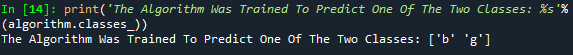```# We view the specific classes the model was trained to predict.

print('The Algorithm Was Trained To Predict One Of The Two Classes: %s'%(algorithm.classes_))```

Output to the above code block will be seen as follows:```print("""The Model Says The Probability Of The Observation We Passed Belonging To Class ['b'] Is %s"""%(algorithm.predict_proba(observation)))
print()
print("""The Model Says The Probability Of The Observation We Passed Belonging To Class ['g'] Is %s"""%(algorithm.predict_proba(observation)))```

The expected output would be as follows: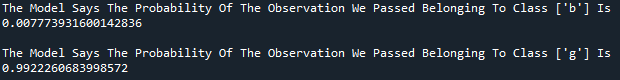## Conclusion.

This concludes my article. We now understand the Logic behind this Supervised Machine Learning Algorithm and know how to implement it in a Binary Classification Problem.